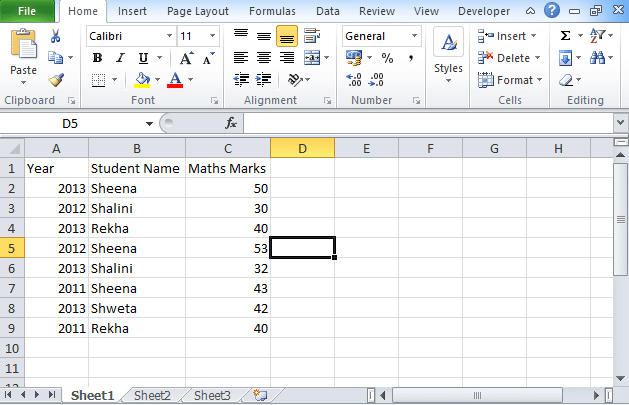To advertise with us contact on Whatsapp: +923041280395 For guest post email at: [email protected]

# How to Use SumIfs Function in Excel [Multiple Criteria]The SumIf function returns the summation of all the numbers who met the particular criteria. You have to use this SUMIF function in Excel which is also known as worksheet function. The function is used to add the value of all the cells depending on date, text, and numbers. It also supports the logical operator and wildcards.

## SumIfs Function in Excel (Syntax)

The syntax of the SumIfs function in excel is

SUMIFS(sum_range, criteria_range1, criteria1, [criteria_range2, criteria2,……., criteria_n)

Where sum_range: The range of cells that you want to sum.

Criteria_range1: The cells range on which you want to apply criteria 1 against.

Criteria1: This determines which cells to add.

Criteria_range2,…..criteria_range_n: In this range of cells you can take up to 127 ranges.

### How to use SumIfs function?

The named range is called a descriptive name used for the collection of cells or you can mention the range. The SumIfs function is similar to the excel If statement multiple conditions range:

• Let’s take a data set of students. Check the above image.
• In column A, list the years and student name’s in column B, and Marks in Maths in column C.
• Now calculate SumIfs in column D.
• The formula for SUMIfs is “=SUMIFs(C2:C9, A2:A9,”=2012”,B2:B9,”=Sheena”)”.
• In this syntax, A2:A9,”=2012” is the criteria 1 whereas B2:B9,”=Sheena” is the criteria 2.
• Now to view named ranges, go to the formulas tab located at the ribbon.
• Look for the defined names.
• Click on the drop-down list.
• Click on the Name Manager.
• Soon after clicking on the Name manager, you will find a new pop-up window.

## Few points to remember using the SUMIFs function

The SUMIFs function can be applied on more than one set of criteria having more than one range.

• Every additional range must have the similar number of rows and columns that are denoted as sum-range.
• You must enclose non-numeric criteria in double quotes whereas the numeric criterion doesn’t require to get enclosed in the double quotes.
• You can use wildcard characters like ? and *in the criteria.
• You can use tilde (~) or asterisk (~?, ~*) to find a literal question.
• In SUMIF and SUMIFs, you can mention only ranges, not arrays.
• The arguments that you mention in SUMIFs and SUMIF are different.

You can also use the sumif function which is quite similar to SUMIFs. The difference between these two is that in SUMIF you can test only one condition whereas, in SUMIFs, it is allowed to test 127 criteria.

## Why use a SUMIFs function instead of SUM function?

The SUMIFs function is used to perform a comparison. To compare values, you need operators like ‘=’, ‘<’, ‘>’. If you want to use these operators, you can apply them to the sum range or the criteria range. You can create the comparison operators using “<=” which is less than or equal to, “>=” is the greater than or equal to sign, and “<>” is the less than or greater than sign.

## SUMIF function

The SumIf function is used to test only a single condition. The syntax of the SUMIF function is:

=SUMIF(range, criteria, [sum_range])

where

Range: The parameter gives you the range of the cells that are evaluated in the criteria parameter.

Criteria: The parameter is the condition that must be met in the range parameter.

Sum-Range: This is an optional parameter. If you are not using Sum_range then it will take the default.